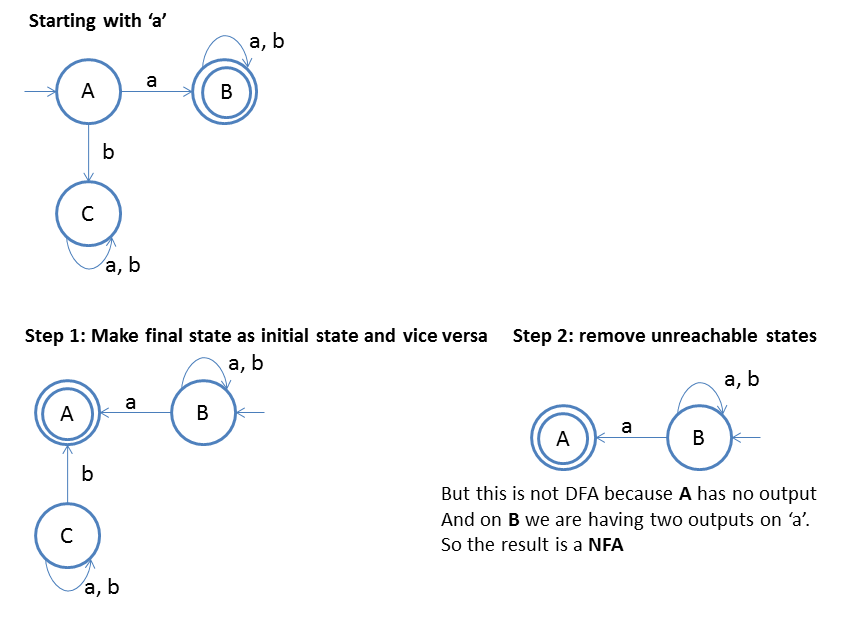## Conversion of Finite Automata to Left Linear Grammar

Converting finite automata to left lienar grammar is somewhat tricky.
See below steps and example followed by it, we will understand the process.

1. Take reverse of the finite automata
2. Then write right linear grammar following the previous lesson
3. Then take reverse of the right linear grammar
4. And you will get the final left linear grammar
Note:If you don't know how to take the reverse of FA then Click HereTake the step 2 output and we will write down "right linear grammar" for it.
Begin from start state, that is B
So,
```			B -> aB/bB/aA
A -> ε
```

And now reverse the right linear grammar to get the ouput = left linear Grammar
```
B -> Ba/Bb/Aa
A -> ε
```

### Formula

FA -> Reverse of FA -> Right Linear Grammar -> Reverse of Right Linear Grammar = Left Linear Grammar